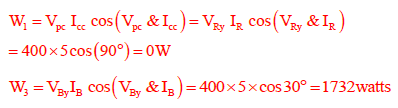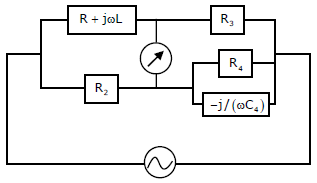# Electrical and Electronic Measurements - Online Test

Q1. Match the following:
Instrument Type                                                                        Used for
P. Permanent magnet moving coil                                            1. DC only
Q. Moving iron connected through current transformer            2. AC only
R. Rectifier                                                                                3.AC and DC
S. Electrodynamometer
Answer : Option C
Explaination / Solution:
No Explaination.

Q2. A 3-phase balanced load which has a power factor of 0.707 is connected to balanced supply. The power consumed by the load is 5kW. The power is measured by the two wattmeter method. The readings of the two wattmeters are
Answer : Option A
Explaination / Solution:satisfiying only for(A)

Q3. A single-phase, 22 kVA, 2200 V/ 220 V, 50 Hz, distribution transformer is to be connected as an auto-transformer to get an output voltage of 2420 V. Its maximum kVA rating as an autotransformer is
Answer : Option C
Explaination / Solution:Q4. The slope and level detector circuit in a CRO has a delay of 100 ns. The start-stop sweep generator has a response time of 50 ns. In order to display correctly, a delay line of
Answer : Option A
Explaination / Solution:

The delay line should be inverted in VDP (Y-channel) only.

Q5. The load shown in the figure is supplied by a 400 V (line to line) 3-phase source (RYB sequence). The load is balanced and inductive, drawing 3464 VA. When the switch S is in position N, the three watt-meters W1W2 and W3 read 577.35 W each. If the switch is moved to position Y, the readings of the watt-meters in watts will beAnswer : Option D
Explaination / Solution:Q6. A wattmeter is connected as shown in the figure. The wattmeter readsAnswer : Option D
Explaination / Solution:
No Explaination.

Q7. An ammeter has a current range of 0-5A, and its internal resistance is 0.2Ω. In order to change the range to 0-25A, we need to add a resistance of
Answer : Option D
Explaination / Solution:
No Explaination.

Q8.
Consider a stator winding of an alternator with an internal high-resistance ground fault. The currents under the fault condition are as shown in the figure. The winding is protected using a differential current scheme with current transformers of ratio 400/5 A as shown. The current through the operating coil isAnswer : Option C
Explaination / Solution:
No Explaination.

Q9. The Maxwell’s bridge shown in the figure is at balance. The parameters of the inductive coil areAnswer : Option A
Explaination / Solution:
No Explaination.

Q10. The pressure coil of a dynamometer type wattmeter is
Answer : Option B
Explaination / Solution:

Since all the applied voltage appears across it.